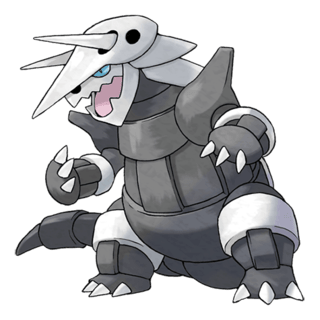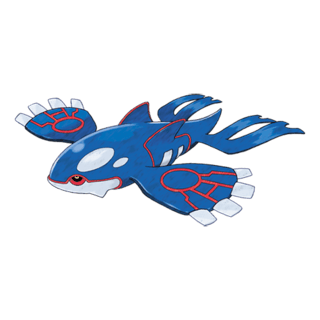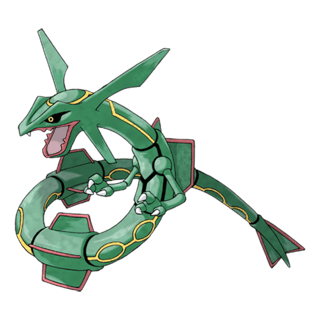# How does Raid Boss CP work?

Have you ever wondered which formula Pokemon GO uses to calculate Raid Bosses CP? Raid Bosses are buffed up versions of regular Pokémon that take over gyms and can provide quite the fight for a group of trainers.

However, on the mechanical aspect, how do they work? How can their CP rise into the tens of thousands and provide the level of challenge they do? What makes a raid boss, a raid boss?

### Raid Boss CP Formula in Pokemon GO

Starting with CP, it far surpasses any normal Pokemon that can be acquired by a trainer. Surprisingly though, the CP formula for raid bosses is actually simpler to calculate that the regular CP formula.

#### Normal Pokemon CP Formula

[(BaseAttack + AttackIV) x (BaseDefense + DefenseIV)1/2 x (BaseStamina + StaminaIV)1/2 x  (CP Multiplier)2] / 10

This is how Pokémon Go calculates the CP of regular Pokemon, with the basics being adding the individual values (IVs) to the base stats of a Pokémon , multiplying it all together after taking the square root (sqrt) of defense and stamina, and then multiplying that number to a number calculated from a designated CP multiplier (chart here courtesy of Gamepress) being squared and divided by ten.

As an example, a perfect Kyogre, with base stats of 270 attack, 251 defense, and 182 stamina, caught from a raid boss is 2328, and can be proven as seen by this completed equation:

((270+15)*sqrt((251+15))*sqrt((182+15)))*((0.59740001^2)/10) = 2328.35

Numbers round down, so after the decimal doesn’t matter. For more info, see this previous Go Hub article.

#### Raid Boss CP Formula

[(BaseAttack + 15) x (BaseDefense+15)1/2 x Stamina1/2] / 10

As stated, Raid Boss CP is much easier to caclulate, as all raid boss CP multipliers are 1, so that part of the equation just gets reduced to the division of 10 at the end. A Raid Boss always has perfect attack and defense IVs, so 15 would go in those slots.

The only change thereafter is stamina, which is set to a static value, not as an IV, and varies by the raid tier. (Original discovery by several Reddit users, including haxxxahClamusChowderus, and vlfph on threads here and here.)

Raid Boss Stamina Values
Raid Tier Stamina Value
Tier 1 600
Tier 2 1,800
Tier 3 3,000
Tier 4 7,500
Tier 5 (Legendary) 12,500

Not only does this allow sense to be made over seemingly arbitrary CP values for the various bosses, but it explains how these values can be known for any and all other Pokémon in simulations – in advance.

Take an actual raid boss, Kyogre again, with its aforementioned stats, and its theoretical follow-up at the time of writing, Rayquaza, with its 284 attack and 170 defense, as well as raid newcomer Aggron, with its tier 4 stamina values and a 198 attack and 314 defense:

Raid Boss CP Calculation
Raid Boss Equation Actual Raid CPAggron ((198+15) * sqrt(314+15) * sqrt(7500)) / 10 = 33458.6322942226674634 33458Kyogre ((270+15) * sqrt(251+15) * sqrt(12500)) / 10 = 51968.5602071098367658 51968Rayquaza ((284+15) * sqrt(170+15) * sqrt(12500)) / 10 = 45468.6499139791921753 45468

## Stats After the Battle

After a raid boss is defeated, the stats get rolled for the boss instance you get to catch, going back to known CP and stat conventions, ranging in value from 67% at 10 attack, 10 defense, and 10 stamina, to 100% with 15 attack, 15 defense, and 15 stamina.

These values are random for every trainer, and generally raid bosses will have guides that denote the top percentile of IVs. (Such as the one found at the bottom of the Kyogre raid guide here.)